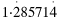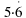What is the meant by "Give the exact answer to:
a) 9 divided by 7 and
b) 1.7 divided by 0.3."

Thanks for this, Jon! The answer is likely to be "in fraction form".

Do 9 ÷ 7 on a calculator and you get 1·2857142857... which is a recurring decimal. So we could write. But this isn't really what it means.

Similarly, 1.7 ÷ 0·3 = 5·666666666666... which is.

What the question really wants is a fractional form:

Firstly, 9/7 is a top-heavy fraction, so we can write it instead as 1 2/7.

Secondly, 1·7/0·3 isn't a valid fraction, so we find an equivalent fraction by multiplying top and bottom by 10: 17/3 = 5 2/3

These are "exact answers". I hope this helps!Fractions TutorialsMaths Menu